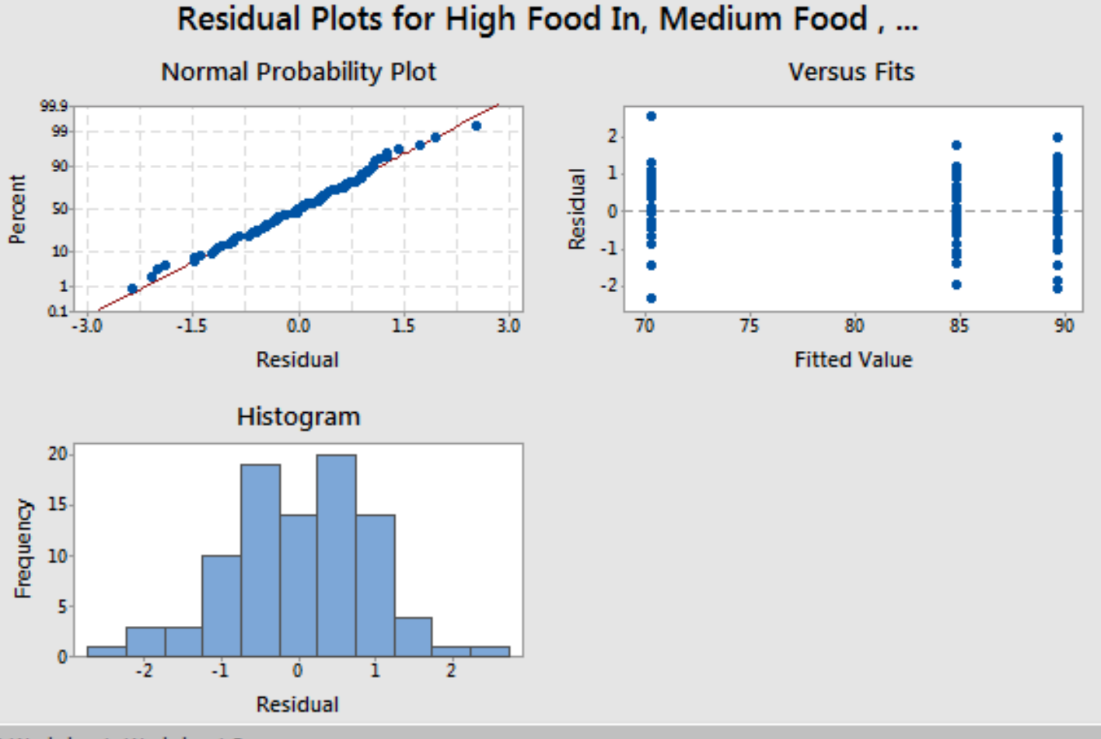# 9.2 - Assumptions for One-Way ANOVA Test

9.2 - Assumptions for One-Way ANOVA Test

If you recall, there were four assumptions for regression (LINE), in ANOVA there are three primary assumptions (NOTE the missing assumption is linearity which actually does not make much sense when working with categorical predictors!):

1. The responses for each factor level have a normal population distribution.
2. These distributions have the same variance.
3. The data are independent.
Note! Violations to the first two that are not extreme can be considered not serious. The sampling distribution of the test statistic is fairly robust, especially as sample size increases and more so if the sample sizes for all factor levels are equal. If you conduct an ANOVA test, you should always try to keep the same sample sizes for each factor level.

With Moriah’s data, we can examine the residual plots to determine if these assumptions are met.As was the case with regression, normality is established by seeing a bell curve in the histogram. Equal variance is reflected in the “Versus Fits” plot, with the spread of the blue dots about the same across all three levels of the fitted values. Finally, independence is determined due to the nature of the study not being constructed of dependent sampling units.

  Link ↥ Has Tooltip/Popover Toggleable Visibility